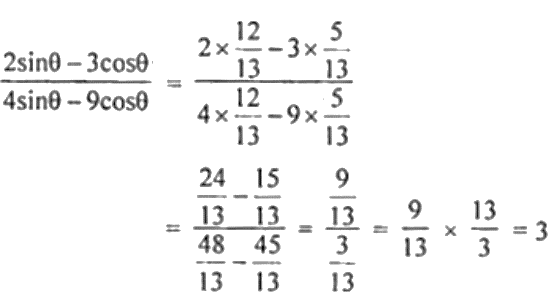Guru

# If 12 cosec θ = 13, find the value of (2 sin θ – 3 cos θ)/ (4 sin θ – 9 cos θ)

• 0

This ques has been taken from the Book- ML Aggarwal
Board- ICSE
Publication- Avichal

Chapter 18th
Chapter- Trigonometric Identities

This has been asked in previous year question

So it is very important ques

If 12 cosec θ = 13, find the value of (2 sin θ – 3 cos θ)/ (4 sin θ – 9 cos θ)

ML Aggarwal, ICSE, class10, chapter 18, question no 5

Share

1. Given,

12 cosec θ = 13

⇒ cosec θ = 13/12

In right ∆ ABC,

∠A = θ

So, cosec θ = AC/BC = 13/12

AC = 13 and BC = 12

By Pythagoras theorem,

AB = √(AC2 – BC2)

= √[(13)2 – (12)2]

= √(169 – 144)

= √25

= 5

Now,

sin θ = BC/AC = 12/13

cos θ = AB/AC = 5/13

Hence,• 0